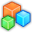# Microsoft Small BasicProgram Listing: DDF986
A = "अ"
A = "आ"
A = "आा"
A = "इ"
A = "ई"
A = "ईी"
A = "उ"
A = "ऊ"
A = "ऊू"
A = "ॠ"
A = "ॠृ"
A = "ॠृा"
A = "ळ"
A = "ळु"
A = "ळू"
A = "ए"
A = "एा"
A = "एाा"
A = "ऎ"
A = "ऐॊ"
A = "ऐॊॊ"
A = "ओ"
A = "ओो"
A = "ओोो"
A = "औ"
A = "औौ"
A = "औौौ"
A = "क्"
A = "ख्"
A = "ग्"
A = "घ्"
A = "ङ्"
A = "च्"
A = "छ्"
A = "ज्"
A = "झ्"
A = "ञ्"
A = "ट्"
A = "ठ्"
A = "ड्"
A = "ढ्"
A = "ण्"
A = "त्"
A = "थ्"
A = "द्"
A = "ध्"
A = "न्"
A = "प्"
A = "फ्"
A = "ब्"
A = "भ्"
A = "म्"
A = "य्"
A = "र्"
A = "ल्"
A = "व्"
A = "श्"
A = "ष्"
A = "स्"
A = "ह्"
A = "ं"
A = "ः"
A = "…"
A = "::"
For I = 1 To 729
' The following line could be harmful and has been automatically commented.
CKR[I] = X
'TextWindow.Write(I + ": " + CKR [I] + " ")
EndFor
' Starting First Chakra Bandh
GraphicsWindow.Title = "Siri Bhoovalaya Chakra 1-1-1"
GraphicsWindow.Width=500
GraphicsWindow.Height=500

IRW = 1
ICL = 14
For l = 1 To 27
RW = IRW
CL = ICL
For J = 1 To 27
PTR = 27*(RW-1) + CL
P = CKR[PTR]
LRW = RW
LCL = CL
GraphicsWindow.DrawText(15*J, 15*l, " " + A[P] )
TextWindow.WriteLine(l + "-" + J + " Row = "+RW + " Column = "+CL + " Code = " + CKR[PTR])
' The following line could be harmful and has been automatically commented.
' File.AppendContents("F:\SmallBasicProgs\OutC111.dat", A[P])
RW = RW - 1
CL = CL + 1
If RW < 1 Then
RW = 27
EndIf
If CL > 27 Then
CL = 1
EndIf

EndFor
IRW = LRW + 1
ICL = LCL
If IRW> 27 Then
IRW = 1
EndIf